# RD Sharma Solutions for Class 8 Math Chapter 3 - Squares and Square Roots (Part-6) Notes | Study RD Sharma Solutions for Class 8 Mathematics - Class 8

## Class 8: RD Sharma Solutions for Class 8 Math Chapter 3 - Squares and Square Roots (Part-6) Notes | Study RD Sharma Solutions for Class 8 Mathematics - Class 8

The document RD Sharma Solutions for Class 8 Math Chapter 3 - Squares and Square Roots (Part-6) Notes | Study RD Sharma Solutions for Class 8 Mathematics - Class 8 is a part of the Class 8 Course RD Sharma Solutions for Class 8 Mathematics.
All you need of Class 8 at this link: Class 8

#### Question 5: Find the square root in decimal form:150.0625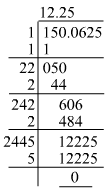Hence, the square root of 150.0625 is 12.25.

#### Question 6: Find the square root in decimal form:225.6004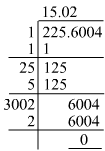Hence, the square root of 225.6004 is 15.02

#### Question 7: Find the square root in decimal form:3600.720036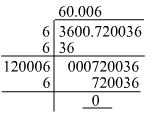Hence, the square root of 3600.720036 is 60.006.

#### Question 8: Find the square root in decimal form:236.144689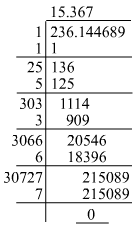Hence, the square root of 236.144689 is 15.367.

#### Question 9: Find the square root in decimal form:0.00059049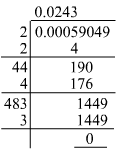Hence, the square root of 0.00059049 is 0.0243.

#### Question 10: Find the square root in decimal form:176.252176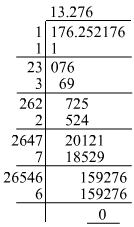Hence, the square root of 176.252176 is 13.276.

#### Question 12: Find the square root in decimal form:0.00038809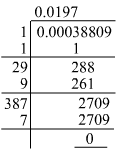Hence, the square root of 0.00038809 is 0.0197.

#### Answer 15:We have to find the square root of the given number.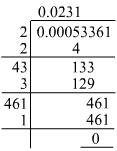Hence, the fraction, which when multiplied by itself, gives 0.00053361 is 0.0231.

#### Question 16: Simplify: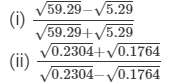#### Answer 16: (i) We have: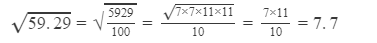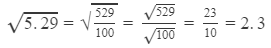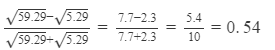(ii) We have: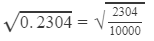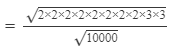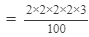=0.48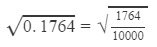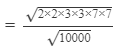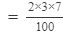=0.42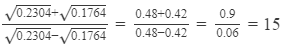#### Answer 17: We have: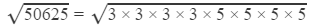=3×3×5×5=225

Next, we will calculate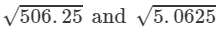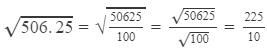=22.5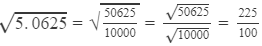=2.25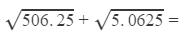22.5+2.25=24.75

#### Question 18: Find the value of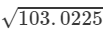and hence find the value of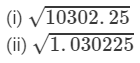#### Answer 18: The value of 103.0225 is:Hence, the square root of 103.0225 is 10.15.
Now, we can solve the following questions as shown below:

(i)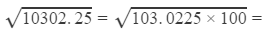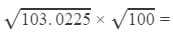10.15×10=101.5

(ii)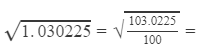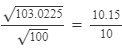=
1.015

#### Question 19: Find the square root of each of the following correct to three places of decimal.(i) 5(ii) 7(iii) 17(iv) 20(v) 66(vi) 427(vii) 1.7(viii) 23.1(ix) 2.5(x) 237.615(xi) 15.3215(xii) 0.9(xiii) 0.1(xiv) 0.016(xv) 0.00064(xvi) 0.019

(xvii) 7/8

(xviii)  5/12

(xix)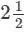(xx)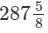#### Answer 19: (i) We can find the square root up to three decimal places by using long division until we get four decimal places and then rounding it to three decimal places.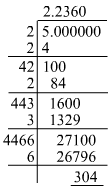Hence, the square root of 5 up to three decimal places is 2.236.
(ii) We can find the square root up to three decimal places by using long division until we get four decimal places and then rounding it to three decimal places.Hence, the square root of 7 up to three decimal places is 2.646.
(iii) We can find the square root up to three decimal places by using long division until we get four decimal places and then rounding it to three decimal places.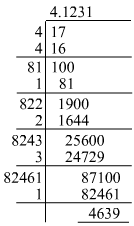Hence, the square root of 17 up to three decimal places is 4.123.
(iv) We can find the square root up to three decimal places by using long division until we get four decimal places and then rounding it to three decimal places.Hence, the square root of 20 up to three decimal places is 4.472.
(v) We can find the square root up to three decimal places by using long division until we get four decimal places and then rounding it to three decimal places.Hence, the square root of 66 up to three decimal places is 8.124.
(vi) We can find the square root up to three decimal places by using long division until we get four decimal places and then rounding it to three decimal places.Hence, the square root of 427 up to three decimal places is 20.664.
(vii) We can find the square root up to three decimal places by using long division until we get four decimal places and then rounding it to three decimal places.Hence, the square root of 1.7 up to three decimal places is 1.304.
(viii) We can find the square root up to three decimal places by using long division until we get four decimal places and then rounding it to three decimal places.Hence, the square root of 23.1 up to three decimal places is 4.806.
(ix) We can find the square root up to three decimal places by using long division until we get four decimal places and then rounding it to three decimal places.Hence, the square root of 2.5 up to three decimal places is 1.581.
(x) We can find the square root up to three decimal places by using long division until we get four decimal places and then rounding it to three decimal places.Hence, the square root of 237.615 up to three decimal places is 15.415.
(xi) We can find the square root up to three decimal places by using long division until we get four decimal places and then rounding it to three decimal places.Hence, the square root of 15.3215 up to three decimal places is 3.914.
(xii) We can find the square root up to three decimal places by using long division until we get four decimal places and then rounding it to three decimal places.Hence, the square root of 0.9 up to three decimal places is 0.949.
(xiii) We can find the square root up to three decimal places by using long division until we get four decimal places and then rounding it to three decimal places.Hence, the square root of 0.1 up to three decimal places is 0.316.
(xiv) We can find the square root up to three decimal places by using long division until we get four decimal places and then rounding it to three decimal places.Hence, the square root of 0.016 up to three decimal places is 0.126.
(xv) We can find the square root up to three decimal places by using long division until we get four decimal places and then rounding it to three decimal places.Hence, the square root of 0.00064 up to three decimal places is 0.025.
(xvi) We can find the square root up to three decimal places by using long division until we get four decimal places and then rounding it to three decimal places.Hence, the square root of 0.019 up to three decimal places is 0.138.
(xvii) We can find the square root up to four decimal places by expanding 7/8 to decimal form up to eight digits to the right of the decimal point as shown below:
7/8=0.87578=0.875
Hence, we have:So, the square root of 7/8 up to three decimal places is 0.935.
(xviii) We can find the square root up to four decimal places by expanding 5/12 to decimal form up to eight digits to the right of the decimal point as shown below: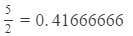Hence, we have:So, the square root of 5/12 up to three decimal places is 0.645.
(xix) We can find the square root up to four decimal places by expanding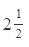into decimal form up to eight digits to the right of the decimal point as shown below:=2.50000000212=2.50000000
But, this is the same with the value 2.5 in problem (ix). Hence, the square root ofis 1.581.
(xx) We can find the square root up to four decimal places by expanding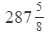28758 into decimal form up to eight digits to the right of the decimal point as shown below:=
287.62500000
Hence, we have:So, the square root ofup to three decimal places is 16.960.

The document RD Sharma Solutions for Class 8 Math Chapter 3 - Squares and Square Roots (Part-6) Notes | Study RD Sharma Solutions for Class 8 Mathematics - Class 8 is a part of the Class 8 Course RD Sharma Solutions for Class 8 Mathematics.
All you need of Class 8 at this link: Class 8Use Code STAYHOME200 and get INR 200 additional OFF

## RD Sharma Solutions for Class 8 Mathematics

88 docs

Track your progress, build streaks, highlight & save important lessons and more!

,

,

,

,

,

,

,

,

,

,

,

,

,

,

,

,

,

,

,

,

,

;